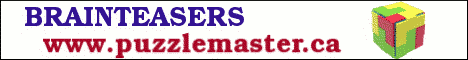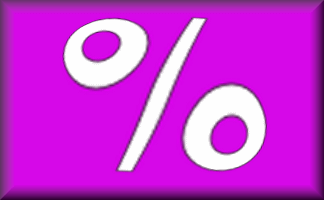#20% of £130

£26

50% of £26

£13

25% of £364

£91

10% of £1000

£100

5% of £1540

£77

10% of £220

£22

20% of £455

£91

5% of £1160

£58

10% of £490

£49

10% of £580

£58

5% of £1480

£74

50% of £98

£49

5% of £2200

£110

25% of £296

£74

20% of £180

£36

10% of £260

£26

10% of £1100

£110

25% of £88

£22

50% of £200

£100

25% of £144

£36

### C

20% of £385

£77

Answers are in pairs. Which is the odd one out?

## A Mathematics Lesson Starter Of The Day

Share

Topics: Starter | Percentages

• Cate, England
•
• Option to change currency please!

[Transum: The option to change currency is now available below. Thanks for the suggestion.]
• McDonald, Oban
•
• Why R? L is the only answer that is a decimal. That is an odd one out? I'll use this as a nice discussion point in my class. Thank you.
• Transum,
•
• It is up to the teacher whether to allow the use of calculators but most of the pupils attempting this starter should be able to work out each of these simple percentages in their heads or with pencil and paper. They should know strategies such as to work out 20% it is easy to find 10% and then double it. A pencil and paper will be needed to keep track of the answers so the odd one out, the one without a partner, can be identified.
• C Clover, UK
•
• Great page - would be even better if interactive so pupils could click on two that match and check they are correct.

How did you use this starter? Can you suggest how teachers could present or develop this resource? Do you have any comments? It is always useful to receive feedback and helps make this free resource even more useful for Maths teachers anywhere in the world.
Click here to enter your comments.

If you don't have the time to provide feedback we'd really appreciate it if you could give this page a score! We are constantly improving and adding to these starters so it would be really helpful to know which ones are most useful. Simply click on a button below:

Excellent, I would like to see more like this
Good, achieved the results I required
Satisfactory
Didn't really capture the interest of the students
Not for me! I wouldn't use this type of activity.

This starter has scored a mean of 2.9 out of 5 based on 454 votes.

Previous Day | This starter is for 16 February | Next Day

% - This is the percent symbol.
Percent means 'out of 100'.

### Find 50%:

As 50 is half of 100, then 50% means half. To find 50% of a quantity you need to halve (or divide by two). So 50% of 6 is 3.

### Find 10%:

As 10 is one tenth of 100, then 10% means 'one tenth of'. To find 10% of a quantity you need to divide it by ten. So 10% of 800 is 80.

### Find 25%:

As 25 is one quarter of 100, then 25% means 'one quarter of'. To find 25% of a quantity you need to divide it by four. So 25% of 20 is 5.

Another way of finding 25% of a quantity is first finding 50% then dividing the result by 2.

### Find 33⅓%:

As 33⅓ is one third of 100, then 33⅓% means 'one third of'. To find 33⅓% of a quantity you need to divide it by three. So 33⅓% of 30 is 10.

### Find 1%:

As 1 is one hundredth of 100, then 1% means 'one hundredth of'. To find 1% of a quantity you need to divide it by 100. So 1% of 800 is 8.

### Find other percentages:

Other percentages can be found by combining some of the techniques mentioned above. Here are some examples:

• To find 75% of a quantity add together 50% and 25% of it.
• To find 20% of a quantity double 10% of it.
• To find 5% of a quantity halve 10% of it.
• To find 66⅔% of a quantity double 33⅓% of it.
• To find 90% of a quantity subract 10% from that quantity.
• To find 80% of a quantity subract 20% from that quantity.
• To find 2% of a quantity double 1% of it
• To find 52% of a quantity add 50% to 2% of that quantity

If you need to use a calculator to check your working. See Calculator Workout skill 3.

### Commute

Did you know that if you are struggling to mentally work out 24% of 50 you can switch the numbers round and work out 50% of 24 instead. Finding 50% is very easy isn’t it? You will get the same answer.

Finding a percentage of a quantity is an example of a commutative calculation. Not all operations are commutative. Subtraction certainly isn’t as 10 minus one is not the same as one minus ten.

You can use this trick to improve your ability to do this type of calculation quickly if you find the switch makes it easier.

Practise with 12% of 50, 4% of 25 and 75% of 10.

## AnswerThis activity is suitable for students of mathematics all around the world. Use the button below to change the currency symbol used to make it more relevant to your students. You may wish to choose an unfamiliar currency to extend your students' experience.Note to teacher: Doing this activity once with a class helps students develop strategies. It is only when they do this activity a second time that they will have the opportunity to practise those strategies. That is when the learning is consolidated. Click the button above to regenerate another version of this starter from random numbers.

Your access to the majority of the Transum resources continues to be free but you can help support the continued growth of the website by doing your Amazon shopping using the links on this page. Below is an Amazon search box and some items chosen and recommended by Transum Mathematics to get you started.Teacher, do your students have access to computers?Do they have iPads or Laptops in Lessons? Whether your students each have a TabletPC, a Surface or a Mac, this activity lends itself to eLearning (Engaged Learning).Here a concise URL for a version of this page without the comments.

Transum.org/go/?Start=February16

Here is the URL which will take them to a related student activity.

Transum.org/go/?to=PercentagesFor All:

©1997-2019 WWW.TRANSUM.ORG